# Adjusting for covariate misclassification in logistic regression – predictive value weighting

When we fit regression models, we implicitly assume that the values in our dataset are accurate measurements of the variables of interest. In many settings, the measurements we actually have are imperfect. In the case of a categorical variable, for some of the records in our dataset the observed value may differ from the true value, due to misclassification. Misclassification arises for many different reasons. In epidemiology, instruments are often used to measure conditions imperfectly – sometimes observations which should be recorded as 1 are recorded as 0, and vice-versa. In this post I’ll focus on the common situation where logistic regression is used to model an outcome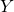, and one of the covariates is subject to misclassification.

Misclassification in a simple model with one binary covariate
First let’s consider the simple (but arguably rare) situation where our model has a single (true) binary covariate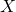. The logistic regression model is then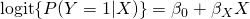Ideally we would obtain a sample of data on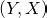, and fit the logistic regression model to estimate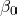and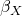.

But let us assume that rather than, our dataset contains a misclassified version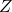. The misclassification mechanism can be characterised by the sensitivity and specificity: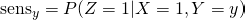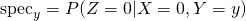The subscript y denotes the fact that the misclassification probabilities may vary depending on the value of the outcome. The misclassification is said to be non-differential (with respect to) if in fact the sensitivity and specificity are the same for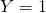and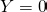.

What happens if we ignore the misclassification, and fit the logistic regression model withas covariate? As one might expect, we will obtain biased estimates – the intercept and coefficient ofwill not be (asymptotically) unbiased estimates ofand.

Can we say anything more about the bias? Well, in the special case where the misclassification is non-differential, and we have no other covariates in the model, the bias in the coefficient ofwill always be towards the null, meaning towards an odds ratio of 1. Another way of putting this is that the association between the covariate and outcome will be diluted (in expectation). It’s important to remember that this result only applies when there is a single binary covariate in the model, and the misclassification is non-differential. With differential misclassification, the effect might actually be to make the association larger than it should.

It is easy to empirically demonstrate this with a small simulation in R. To (largely) remove the effects of sampling variability, we’ll simulate data for a study of 100,000 observations. First we simulate thedata, and fit the logistic regression model usingas covariate:

set.seed(54894)
n <- 100000
x <- 1*(runif(n)<0.5)
pr <- exp(x)/(1+exp(x))
y <- 1*(runif(n)|z|)
(Intercept) -0.002346   0.008917  -0.263    0.793
x            0.989095   0.013463  73.470   <2e-16 ***
---
Signif. codes:  0 ‘***’ 0.001 ‘**’ 0.01 ‘*’ 0.05 ‘.’ 0.1 ‘ ’ 1

(Dispersion parameter for binomial family taken to be 1)

Null deviance: 133455  on 99999  degrees of freedom
Residual deviance: 127860  on 99998  degrees of freedom
AIC: 127864

Number of Fisher Scoring iterations: 4


As we should expect, given the sample size is large, the estimates are close to the true parameter values used to simulate the data. In particular, the coefficient ofis close to 1.

Now let's generate the misclassified variable, using a sensitivity of 0.9 and specificity of 0.8, assumed to be the same irrespective of. This can be concisely achieved with the line

z <- (x==1)*(runif(n)<0.9) + (x==0)*(runif(n)<0.2)


Lastly, we refit the model forusingas the covariate:

summary(glm(y~z, family="binomial"))

Call:
glm(formula = y ~ z, family = "binomial")

Deviance Residuals:
Min       1Q   Median       3Q      Max
-1.5254  -1.2169   0.8655   0.8655   1.1384

Coefficients:
Estimate Std. Error z value Pr(>|z|)
(Intercept) 0.092417   0.009418   9.813   <2e-16 ***
z           0.696460   0.013177  52.854   <2e-16 ***
---
Signif. codes:  0 ‘***’ 0.001 ‘**’ 0.01 ‘*’ 0.05 ‘.’ 0.1 ‘ ’ 1

(Dispersion parameter for binomial family taken to be 1)

Null deviance: 133455  on 99999  degrees of freedom
Residual deviance: 130627  on 99998  degrees of freedom
AIC: 130631

Number of Fisher Scoring iterations: 4


The estimated log odds ratio coefficient ofis 0.70, considerably smaller than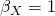- this is a manifestation of the bias caused by the misclassification.

For more on misclassification in this simple one covariate setting, I'd recommend looking at Rothman and Greenland's book, Modern Epidemiology.

The effects of misclassification in a model with multiple covariates
Now let's consider the more realistic situation, where our model contains additional covariates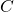: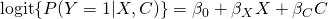As before, let's assume that rather than, we have only a misclassified version. In their most general form, the sensitivity and specificity could now potentially vary as a function both ofand the other covariates, but for simplicity I shall assume they at most depend on the value of.

As before, if we ignore the fact thatis a misclassified version of, and fit our model, our estimates ofandwill be biased. Importantly, in this multiple covariate setting, it is more difficult to predict the direction of biases in the parameter estimates, even when the misclassification is non-differential. In particular, the bias in the coefficient of one of thevariables could be away from the null, if positive confounding is taking place.

Again, we can easily empirically demonstrate this in R with a simulated dataset. To our earlier code, we now add a covariate. I have chosen to simulate it as binary, and to be positively with, and to set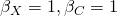:

set.seed(54894)
n <- 100000
x <- 1*(runif(n)<0.5)
c <- 1*(x==1)*(runif(n)<0.7) + 1*(x==0)*(runif(n)<0.3)
pr <- exp(x+c)/(1+exp(x+c))
y <- 1*(runif(n)|z|)
(Intercept) 0.009747   0.010075   0.967    0.333
x           0.996475   0.016151  61.699   <2e-16 ***
c           1.001885   0.016150  62.035   <2e-16 ***
---
Signif. codes:  0 ‘***’ 0.001 ‘**’ 0.01 ‘*’ 0.05 ‘.’ 0.1 ‘ ’ 1

(Dispersion parameter for binomial family taken to be 1)

Null deviance: 121615  on 99999  degrees of freedom
Residual deviance: 108998  on 99997  degrees of freedom
AIC: 109004

Number of Fisher Scoring iterations: 4


Again we obtain estimates which are close to the true parameter values. If we now misclassifyagain, and fit the model usingandas covariates, we obtain:

z <- 1*(x==1)*(runif(n)<0.9) + 1*(x==0)*(runif(n)<0.2)
summary(glm(y~z+c, family="binomial"))

Call:
glm(formula = y ~ z + c, family = "binomial")

Deviance Residuals:
Min       1Q   Median       3Q      Max
-1.9997  -1.1950   0.5394   0.9135   1.1599

Coefficients:
Estimate Std. Error z value Pr(>|z|)
(Intercept)  0.04136    0.01078   3.837 0.000125 ***
z            0.61679    0.01496  41.216  < 2e-16 ***
c            1.19582    0.01556  76.852  < 2e-16 ***
---
Signif. codes:  0 ‘***’ 0.001 ‘**’ 0.01 ‘*’ 0.05 ‘.’ 0.1 ‘ ’ 1

(Dispersion parameter for binomial family taken to be 1)

Null deviance: 121615  on 99999  degrees of freedom
Residual deviance: 111230  on 99997  degrees of freedom
AIC: 111236

Number of Fisher Scoring iterations: 3


For the particular setup I've used here, we see that the log odds ratio coefficient of(standing in for) is biased towards the null. But for the coefficient of, the bias is away from the null. This has occurred becauseandare positively correlated, and have independent positive effects on the log odds of a positive outcome.

Adjusting for misclassification - predictive value weighting
Given that misclassification causes bias in parameter estimates if ignored, what can we do? The first point to make is that in order to allow for misclassification, we need some information about the misclassification. Sometimes this comes in the form of internal validation data, meaning that some of our dataset contain observations of, as well as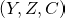. This enables us to estimate the sensitivity and specificity parameters , and make a correction (using some statistical method).

In this post I'll focus on a different situation, where we don't have data with which to estimate the sensitivity and specificity. Instead, we can choose plausible values for these parameters based on our contextual knowledge, and obtain estimates of our parameters of interest (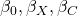which allow for the misclassification. To perform a sensitivity analysis, one can repeat the analysis using different (plausible) values of the sensitivity and specificity.

One approach to doing this which I have found particularly attractive is the predictive value weighting approach, proposed by Lyles and Lin in 2010 (see here for their open access paper). The predictive value weighting approach for our setting consists of the following steps:

1) choose sensitivity and specificity values, possibly dependent onand/or2) specify a logistic regression model for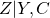3) based on the values chosen in 1) and the fitted model in 2), calculate, for each observation, the predictive value probability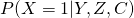, given each observations values of,and4) duplicate each observation in the dataset, and add a variable for the unobserved, with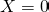in the original record and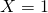in the duplicate record

5) fit a weighted logistic regression model forusing(now available in the dataset) and, with weights given by the predictive values calculated in 3)

For details of the actual calculations in 3), see Lyles and Lin's paper. To obtain valid confidence intervals and tests, we may must be careful not to just use the usual values reported when fitting the model in step 5), since this ignores the impact of the preceding initial steps required to generate the expanded dataset. Lyles and Lin recommend using the jackknife for inference, but bootstrapping is also possible (although Lyles and Lin report experiencing problems with the bootstrap here).

In addition to specifying the sensitivity and specificity values, the key input required by the analyst is choosing the model for. Standard model selection techniques can be used to attempt to ensure it is correctly specified. If the model is mis-specified, estimates will in general still be biased.

Predictive value weighting in R
Implementing predictive value weighting is fairly easy to do. In an R program (file here) I've implemented it in a function called, pvw, for the particular setup used in the simulation earlier. The file also contains code which performs a simulation study. Data are repeatedly sampled from the same setup as used previously, and then the pvw function is called. Note that in this program we are choosing sensitivity and specificity values which match the true values being used to simulate the data. In practice of course we do not know the true sensitivity and specificity values! Reassuringly, the simulation study shows that predictive value weighting successfully recovers unbiased estimates of the parameters.

For inferences, the pvw program could be passed to the boot function in the boot package.

Predictive value weighting in Stata
I have also implemented predictive value weighting in Stata. The program allows sensitivity and specificity values to be specified, possibly differing for cases and controls, but assumed to be the same for different values of. The program can be downloaded from the SSC archive - see the web page here for details. It can be installed in Stata by typing:

ssc install pvw


in the command window.

Probabilistic bias analysis and Bayesian approaches
A different approach to performing a sensitivity analysis is to take a Bayesian approach, where we specify prior distributions for the unknown sensitivity and specificity parameters. We then obtain a single point estimate for our parameters of interest, and credible intervals which are wider to reflect our uncertainty about the sensitivity and specificity parameters. For more on this, and so called 'bias analysis' more generally, I'd recommend the book 'Applying quantitative bias analysis to epidemiologic data', by Lash, Fox and Fink.

This site uses Akismet to reduce spam. Learn how your comment data is processed.